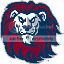-=+=- -=+=- -=+=- -=+=- -=+=- -=+=- -=+=- -=+=- -=+=- -=+=- -=+=- -=+=- -=+=- -=+=- -=+=- -=+=- -=+=- -=+=- -=+=- -=+=- -=+=- -=+=- -=+=- -=+=- -=+=- -=+=- -=+=- -=+=- -=+=- -=+=- (c) WidthPadding Industries 1987 0|721|0 -=+=- -=+=- -=+=- -=+=- -=+=- -=+=- -=+=- -=+=- -=+=- -=+=- -=+=- -=+=- -=+=- -=+=- -=+=- -=+=- -=+=- -=+=- -=+=- -=+=- -=+=- -=+=- -=+=- -=+=- -=+=- -=+=- -=+=- -=+=- -=+=- -=+=- Socoder -> Cobra -> No cobra book :( Posted : Friday, 26 October 2007, 05:29 lyonskrylar stoped working on the book :'( i got the offical word from an e-mail enquiering about it to krylar. a big blow for cobrakrylarHiya, Unfortunately there is no longer a plan for me to write a book for Cobra. Sorry about that.-=-=-Posted : Friday, 26 October 2007, 06:10 power mouseyheya lyonsit could be a big blow to Cobra. And even if it was...... don't cha worry bro! I'm here and we Cobra users and programmers can pool together and especially with both Graham and the Squeaky Duckand learn and share together. True! here is a simple code snippet on Shadow Planes. And I'm not talking about Stargates nor interdimension travel either.Think of a 2d background within 3D and can be moved,transformed, rotated too. And also manipulated thru the camera and lighting too. And also attributes which can be changed and modified such as width, height, xtiles, ytiles, etc. //program name: back3d2.cps program uses cobra3d, keyset var mat1, cam1, lite:element backgr: element txt1,txt2:element camzp:real Begin openscreen camzp=37.75 //load in texture material mat1=CreateTextureMaterial("heroes2.bmp") //create default camera and light cam1=createcamera lite=createlight //position the camera and use camera //repositionthe light...lite UseCamera(cam1) PositionEntity(cam1, 0,0, camzp) PositionEntity(lite, 0,0, 75.0) RotateEntity(lite, 45.0, 0, 0) //create a Shadow Plane...backgr backgr=CreateShadowPlane // change or set some of the Planes' attributes EntityAttribute(backgr, "width", 800) EntityAttribute(backgr, "height", 600) EntityAttribute(backgr, "xtiles", 7) EntityAttribute(backgr, "ytiles", 7) //wrap the texture mat1 onto plane EntityUseMaterial(backgr, mat1) //set the SHadow light.... SetShadowLight(backgr, lite) //main game loop..... Repeat Flip Pause(5) Until KeyDown(VK_ESCAPE) CloseScreen End --v the txt1,txt2 variables are of Element type and will be used later for HUDs texts. hold your head (and mane) up dude and cheerspower mousey